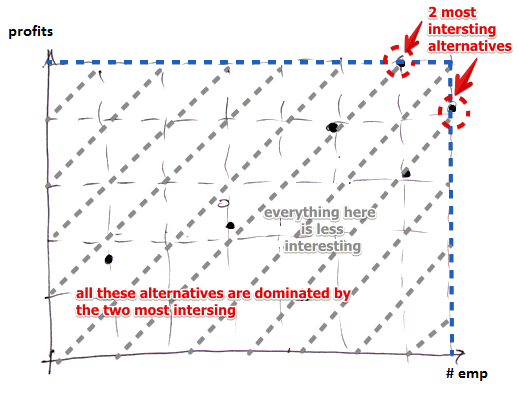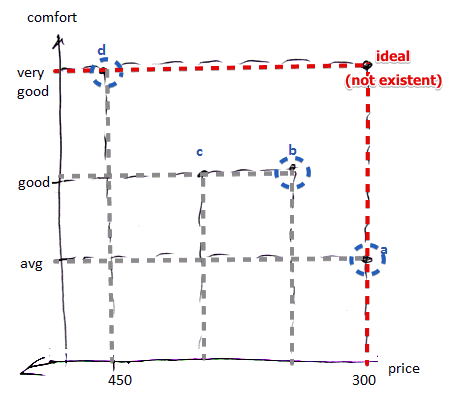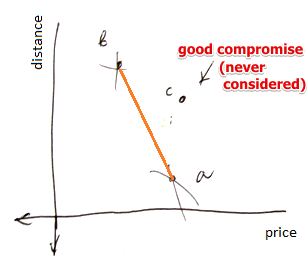# ML Wiki

## Unanimity

Unanimity is principle from Voting Theory that is the same as Dominance:

If a candidate $a$ is always preferred by the majority to $b$, then we can say that $b$ is dominated by $a$ and never consider $b$ again

## Pareto-Optimal Solutions

In Multi-Objective Optimization and Multi-Criteria Decision Aid there could be many "best" solutions - these solutions are called the Pareto-Optimal solutions

Consider this example:

•• the two alternatives along the blue line form the pareto-optimal set
•• the solutions in blue circles also form the pareto-optimal set

Dominance

• for the second examples we can say that $b$ dominates $c$:
• $b$ has the same level of quality, but it is cheaper
• we can remove all dominated solutions from the solution space and this will give us the Pareto-optimal set of solutions
• in MOO this is also called the set of efficient solutions
dominance
$a$ dominates $b$ $\iff \forall i: f_i(a) \geqslant f_i(b)$ and $\exists i: f_j(a) > f_j(b)$
i.e. for all criteria $a$ is at least as good as $b$, but there's at least one criteria at which $a$ is strictly better than $b$

This is not always good. Consider this example

• you're looking for an apartment to rent
• you consider price and distance to work (want to minimize both)
•• in this case $c$ is dominated by $a$ and $b$:
• $b$ is very cheap, $a$ is very close
• $c$ is a good compromise, but it's dominated

Another example

• suppose we're choosing a car
• there are 4 criteria: price, power, consumption, comfort
• there are 6 alternatives
Price Power Consumption Comfort
Avg A. 18 75 8 3
Sport 18.5 110 9 2
Avg B. 17.5 85 7 3
Lux 1 24 90 8.5 5
Exonomic 12.5 50 7.5 1
Lux 2 22.5 85 9 4

We see that Avg B is always better than Avg. A

• then nobody will ever choose Avg A: A is dominated by B

No other alternative can be eliminated this way

## Game Theory

This principle is as well applied in the Game Theory

Notation:

• $A_i$ - set of strategies for player $i$
• $u_i$ - the utility function of $i$
• $a, b \in A_i$ - two strategies in $A_i$
• denote $A_{-i}$ as the set of all strategies for other players
Strict dominance
$a$ strictly dominates $b$ if $\forall c \in A_{-1}: u_i(a, c) > u_i(b, c)$
in other words: $a$ strictly dominates $b$ is for every action that other players can take, the action $a$ gives $i$ better payoff than $b$
Weak dominance
$a$ (weakly) dominates $b$ if $\forall c \in A_{-1}: u_i(a, c) \geqslant u_i(b, c)$

If $a$ dominates all other strategies $b$ of the player $i$ then it's dominant

### Pareto Optimality

In Game Theory there's also a notion of Pareto Optimality

Suppose you see a game as an outside observer, not a player

• Can we say that one outcome $O$ is better than some other outcome $O'$?

Pareto-Dominance

• suppose there's one outcome $O$ that is as good as some other outcome $O'$ for all players
• but there's one agent $i$ who strictly prefers $O$ to $O'$
• then $O$ is considered better than $O'$
• and $O$ pareto-dominates $O'$

Pareto-Optimality

• outcome $O^*$ is pareto-optimal if there is no other outcome that pareto-dominates it
• a game can have more than one pareto-optimal outcome
• for Zero-Sum Games every outcome is pareto-optimal

## Problems

### Close Contenders

$b$ is a close contender if

• it's dominated by some alternative $a$
• but $b$ is still a good choice

Consider this example:

• $A = \{a, b, c, d\}$
• $A^* = \{a, c, d\}$ - efficient (pareto-optimal) set of solutions
$c$ $e_1$ $e_2$ $e_3$ $...$ $e_{100}$
$a$ 100 100 100 ... 100
$b$ 99 99 99 ... 99
$c$ 101 0 0 ... 0
$d$ 0 101 0 ... 0

But we see that $c$ and $d$ aren't that good, but both are in $A^*$

• i.e. we should have taken $b$ instead them